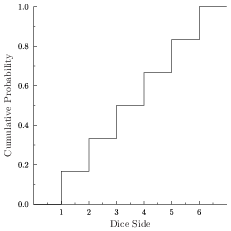# Monte Carlo Simulation

Monte Carlo (MC) systems use random numbers to sample known probability distributions. In each MC calculation, one of more random numbers are generated, and these are used to select from a range of outcomes, depending on the probability of each outcome. This technique allows the simulation of events where the result can be described by cumulative distribution functions (CDFs), which represent probabilities of the outcome of a given event. The results obtained by MC methods are statistical in nature, though the uncertainty associated with a result is only a function of the quantity of sampling. It can be the most accurate method of obtaining a result where a deterministic algorithm cannot be used (Kalos and Whitlock 2008). This sampling approach has existed for over a century, but only achieved significant use with the development of computers and associated tools such as pseudo-random number generators.

### Distribution Functions

CDFs are generally denoted using F(x) and define the probability that a random variable is less than or equal to real number x. For a continuous probability distribution the CDF is defined as (Nelson et al, 1985):$Pr \left\{ \hat{x} \leq x \right\} = F(x) = \int^{\hat{x}}_{-\infty} f(x)\ dx,$

where f(x) is called the probability density function (PDF). F(x), by definition, must have a value between 0 and 1:$\lim_{x \to - \infty} F(x) = 0, \lim_{x \to \infty} F(x) = 1$

For a continuous distribution the probability associated with the random variable is defined as$Pr \left\{ a \leq \hat{x} \leq b \right\} = F(b) - F(a) = \int^b_a f(x)\ dx,$

In the discrete case of a dice roll (seen below in Figure 1), the probability for scoring a 5 can be expressed (assuming the CDF is right-continuous) as$Pr \left\{ \hat{x} = 5 \right\} = Pr \left\{ 5 \leq \hat{x} \leq 6 \right\} = F(6) - F(5) = 1/6$Figure 1: Cumulative distribution function for a dice roll, where the probability of a given side, s, being rolled is f(s) = 1/6.

For a continuous distribution the PDF is the derivative of the CDF and must satisfy$\int^{\infty}_{-\infty} f(x)\ dx = 1.$

### Statistical Sampling

Figure 1 also serves as a tool to explain statistical sampling. To ‘simulate’ a dice roll, a random number, r, in the range 0 to 1 needs to be generated. A value of 0.8, for example, for F(x) in Figure 1 can be mapped to a dice side of 5, so the result of the simulated die roll would be a 5.

Various methods are available for sampling probability distributions: of importance are inverse-transform (or direct method) sampling, the composition method and rejection sampling.

Inverse-transform sampling requires that the CDF can be inverted, either analytically or algorithmically, in which case$\hat{x} = F^{-1}_X (R),$

where R is a random number selected from a uniform distribution from 0 to 1. It should be noted that this method is not necessarily the best option even when the transform exists in an explicit form (Rubinstein and Kroese, 2007). This is the method that was used in the dice roll example above.

The approach generally used when dealing with more complex probability density functions is a mixture of composition and rejection methods (Nelson et al, 1985; Agostinelli et al, 2003). In rejection sampling the dimensions of a bounding box for the PDF are determined, such that a and b satisfy$Pr \left\{\hat{x} < a \right\} = 0 \mathrm{\ and\ } Pr \left\{\hat{x} > b \right\} = 0,$

and c is the largest f(x) in the interval a to b (Rubinstein and Kroese, 2007)$c = sup \left\{ f(x) : x \in \left[a,b\right] \right\}.$

A sample is accepted if, for random numbers r1 and r2 uniformly distributed in intervals a to b and 0 to c respectively,$r_2 \leq f(r_1),$

that is, if the point (r1, r2) lies under the curve f(x). Otherwise the sampled value is rejected and another pair of random numbers (r1, r2) are generated. The efficiency of the method is dependent on the PDF: if there are regions of low probability in the interval a to b rejection will occur more often.

This problem can be mitigated providing the PDF can be expressed as a mixture of PDFs, in the form of (Nelson et al, 1985)$F(x) = \sum^n_{i=1}{\alpha_i f_i(x) g_i(x)}$

where α>0, fi(x) are component PDFs and gi(x) ∈ [0,1]. Here can be sampled by (Nelson et al, 1985; Agostinelli et al, 2003):

• selecting a random integer i ∈ {1,2,…,n} with probability proportional to αi,
• selecting a value x0 from the distribution fi(x),
• calculating gi(x0) and accepting x = x0 with probability gi(x0),
• and if x0 is rejected then the process restarts from step 1.

The quality of this approach depends on the decomposition of the principal PDF: the simpler it is to sample sub-distributions fi(x) and evaluate rejection functions gi(x), the better the method (Agostinelli et al, 2003). Removing the rejection checks (that is, ensuring all gi=1) results in the composition method.

### References

• Agostinelli S et al, 2003. GEANT4 – a simulation toolkit, Nuclear Instruments and Methods in Physics Research A, 506(3): 250-303.
• Kalos M H and Whitlock P A, 2008. Monte Carlo Methods.
• Nelson W R, Hirayama H and Rogers D W O, 1985. The EGS4 Code System.
• Rubinstein R Y and Kroese D P, 2007. Simulation and the Monte Carlo Method.